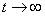Reports of Enlarged Session of the Seminar of I. Vekua

Institute of Applied Mathematics

Volume 22,  2008ISSN 1512-0066

Editional board:

G. Akhalaya, A. Arsenashvili, D. Gordeziani, G. Jaiani, T. Jangveladze (Editor), A. Kharazishili,

S. Kharibegashvili, N. Khomasuridze, Z. Kiguradze (Meneging Editor), A. Kipiani, G. Kipiani,## C O N T E N T S

Abramidze E. On One Variant of Axisymmetric Nonlinear Deformation of  Shells of Revolution Under     Supercritical Load. (pdf file)

Adamia R.  Algorithms For Calculation of Optimal Dynamical Processes and Rational Designing of Machine Systems. (pdf file)

Aleksidze L., Zerakidze Z.  About Strongly and Weakly Separable  Statistical Structures. (pdf file)

Aptsiauri M., Jangveladze T., Kiguradze Z.  On the Stabilization of Solution asand Convergence of the

Corresponding Finite Difference Scheme for One Nonlinear Integro-Differential Equation. (pdf file)

Chokoraia D. On One Mixed Boundary Value Problem for the Non-shallow Spherical Layer. (pdf file)

Ckhvedadze R., Kipiani G., Todua N. Calculation of Rectangular  Double Curvature Flat Shell by Trigonometrical Functions. (pdf file)

Gabrichidze G. New Mathematical Model of Movement of Constraint Particle. (pdf file)

Gedenidze Z., Kvitsiani T., Avaliani S. Optimization Problem of the Cylindrical Covering of the Underground Construction.  (pdf file)

Giorgadze G.  The Linear System of Differential Equations with Deviating  Arguments. (pdf file)

Jaiani G. On a Class of Special Functions. (pdf file)

Jangveladze T., Kiguradze Z., Nikolishvili M.  On  Investigation and Numerical Solution of One Nonlinear  Biological Model. (pdf file)

Jangveladze T., Lobjanidze G. Variational Formulation of One Nonlocal Problem for Forth Order  Ordinary Differential Equation.  (pdf file)

Jikidze L., Tsutskiridze V.  The Steady MHD-Flow of a Low Conductive Fluid in the Neighbourhood of an Infinite Porous Plate at Simultaneous

Rotation of a Plate and Fluid with Strong Magnetic Field.

Kapanadze D.  On the Existence of Solution to Non-local Boundary Problem.  (pdf file)

Kipiani A.  On Cardinalityes of Automorphism Groups of Uniform Binary Relation. (pdf file)

Lomidze I., Javakhishvili J.  Application of the Orthogonal Invariants of Three-dimensional Operators in Some Hydrodynamical Problems. (pdf file)

Manelidze G., Papukashvili A.  About the Use of an Approach Alternative to the Asymptotic Method for Linear        Nonhomogeneous Boundary Problems. (pdf file)

Mania M., Tevzadze R.- Hedging Under Partial Observation. (pdf file)

Meunargia T. On the Complex Representations for the Nonlinear and Non-shallow Shells. (pdf file)

Nadaraya E., Sokhadze G. On the Nonparametric Estimation of a Logarithmic Derivative of a Probability Measure. (pdf file)

Peradze J., Papukashvili G. On One Method of the Solution of a Nonlinear Integro-Differential Equation for a String.  (pdf file)

Purtukhia O., Jaoshvili V. Stochastic Integral Representation of Two-dimensional Poisson

Functionals. (pdf file)

Sharikadze J., Mshvenieradze Kh.  Magnetic Boundary Layer of Second Kind with Strong Suction. (pdf file)

Sokhadze G., Tkeshelashvili A. On One Cylindrical Type Transformation of a Random Measure. (pdf file)

Tsintsadze Z. The Lagrange  Principle of Taking Restrictions off in  Quasilinear Control Systems with Delays.

(pdf file)

Tsitskishvili A., Tsitskishvili R. On the Impact of Two Axially Symmetric Spatial Streams. (pdf file)

Tsutskiridze V., Jikidze L. On the Unsteady Motion of a Viscous Hydromagnetic Fluid Contained Between Rotating  Coaxial Cylinders of Finite Length. (pdf file)

Tvalchrelidze A., Kipiani P. Modeling Exercise of the Plasma Cutting Process. (pdf file)

Valishvili N. Observation on Numerical Solution of Non Linear Equations  System. (pdf file)

Vashakmadze T. To Von Karman Type Systems of Equations for Porous, Piezo and Visco-Elastic Plates. (pdf file)

Zerakidze Z. On Some Properties of Statistical Structure. (pdf file)

Zirakashvili N. The Elastic Equilibrium of Infinite Plate Containing Radial Cracks Originating at the Boundary of Internal Circular Hole. (pdf file)